+---------------------------+
|.-------------------------.|
|| kee_reel@blog:~/en \$ cd ||
|| linux c                 ||
||                         ||
||                         ||
|.-------------------------.|
+-::---------------------::-+
.---------------------------.
// /oooooooooooooooooooooo\\ \\
// /oooooooooooooooooooooooo\\ \\
//-------------------------------\\
\\-------------------------------//

# C. Indexes

A pointer is a variable that stores the address to some memory location.

Usually, everyone is afraid of C and C ++ because of pointers - they seem to be something incomprehensible and very close to how a computer works.

However, it seems to me that even such a complex concept can be explained with real life analogies, which will greatly simplify understanding.

# Looking for treasure

Imagine that you are a treasure hunter - without which no treasure hunt can do? That’s right, no map!

Situation - you have a map on which the coordinates of the treasure are marked, for example: 59.606067, 30.441917.

And now we draw an analogy with the C language:

• Treasure – variable
• Map – Pointer

That is, a map with the coordinates of the treasure is a pointer with the address of a variable.

Let’s create a pointer to a variable:

``````// Ordinary int variable
int treasure = 777;
// Pointer to an int variable (this is indicated by * after the int type)
int* treasure_map;
// Write the address of the int variable treasure to the treasure_map pointer
treasure_map = &treasure;
printf("treasure: %d\n", treasure);
// treasure: 777
printf("coordinates of treasure: %p\n", &treasure);
// coordinates of treasure: 0x7ffe461b4bec
printf("coordinates in treasure_map: %p\n", treasure_map);
// coordinates in treasure_map: 0x7ffe461b4bec
``````

Let’s see what the program outputs:

• “treasure: 777” – the value of our variable (treasure)
• “coordinates of treasure: 0x7ffe461b4bec” – address of variable (hoard coordinates)
• “coordinates in treasure_map: 0x7ffe461b4bec” – pointer value (coordinates written in the map)

Why does the address look like this?

# Address and number representation in memory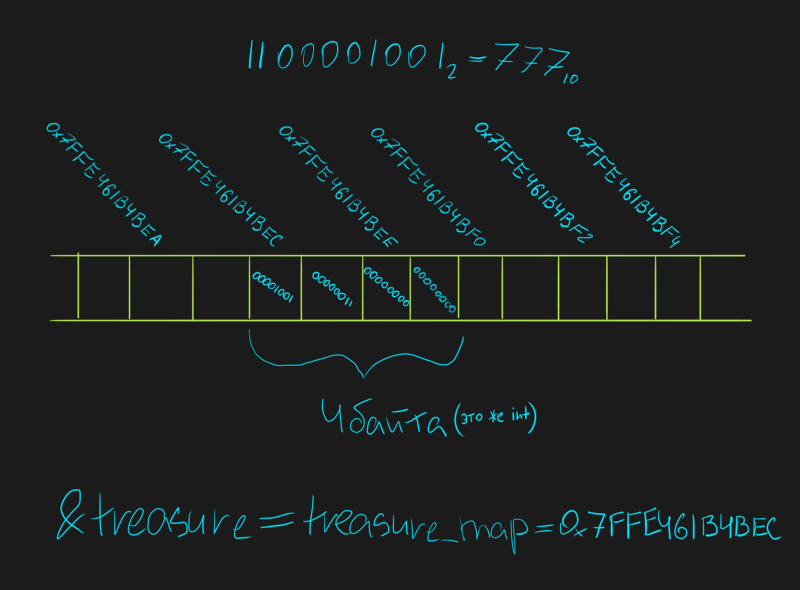You can think of an address as a cell number inside your stick of RAM. Each cell there occupies exactly 1 byte.

For example, I have 16 GB of RAM, which means 16(GB) * 1024(MB) * 1024(KB) = 16777216 bytes. This means that the possible addresses are between 0 and 16777215, or in hexadecimal between 0x0 and 0xFFFFFF.

In my example, the address is greater than 0xFFFFFF, because in fact everything is a little more complicated. I will not explain about memory virtualization, so take this point for now in a simplified form, as I explained above. But if you are very interested, then here is the page on [wikipedia] (https://ru.wikipedia.org/wiki/%D0%92%D0%B8%D1%80%D1%82%D1%83%D0%B0% D0%BB%D1%8C%D0%BD%D0%B0%D1%8F_%D0%BF%D0%B0%D0%BC%D1%8F%D1%82%D1%8C).

Also, you may have noticed that in my example, the number in the cells is written somehow backwards - first there are values, and then zeros, although on a piece of paper we would write this binary number the other way around.

It’s all about the byte order in the number entry: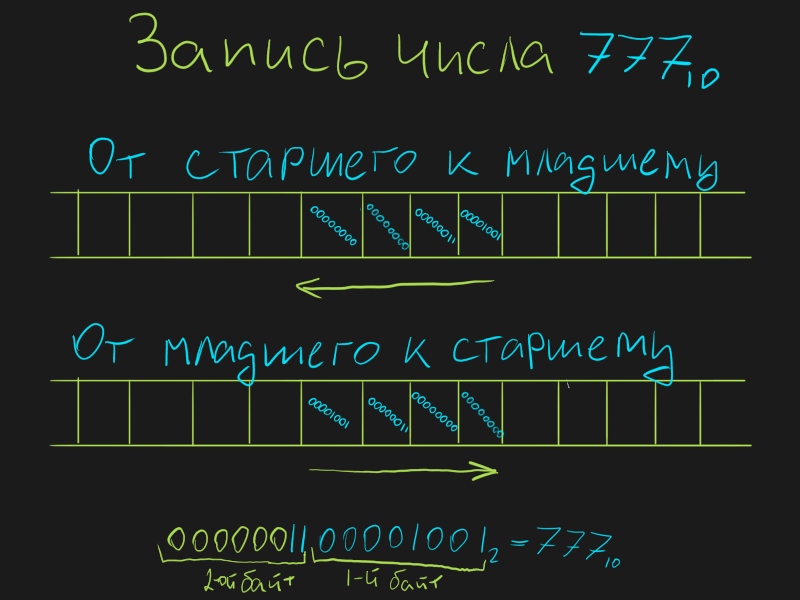Depending on the processor, the byte order you can have is:

• From high to low (big-endian) – the first byte of the number stores the high bits, and the last low bits
• Low to high (little-endian) – the first byte of the number stores the low bits, and the last high bits

A little later in the article we will see live how this manifests itself.

### Pointer size

You probably remember that:

• char takes 1 byte
• short int takes 2 bytes
• float takes 4 bytes
• double takes 8 bytes

So - any pointers take 4 bytes or 8 bytes:

``````int* x;
printf("size of pointer: %d\n", sizeof(x));
// Output: size of pointer: 8
``````

It takes 8 bytes for me. What determines how many bytes a pointer will occupy?

In 32-bit operating systems, working with RAM up to 4 gigabytes is supported - these are addresses from 0 to 4294967295. This number of addresses fits perfectly into 4 bytes - therefore, the pointer takes 4 bytes to fit the address to any cell in mind.

In 64-bit systems, the pointer fits into 8 bytes - here you can already distribute addresses to petabytes of memory.

# Pull out the treasure

Why look at this map - let’s go to the coordinates indicated on the map and dig up the treasure.

Applying this analogy to C, we can get the value of a variable whose address is stored in a pointer:

``````int treasure = 777;
int* treasure_map = &treasure;
printf("coordinates in treasure_map: %p\n", treasure_map);
printf("treasure by coordinates in treasure_map: %d\n", *treasure_map);
/* Conclusion:
coordinates in treasure map: 0x7ffe461b4bec
treasure by coordinates in treasure_map: 777 */
``````

That is, to get the value at the address stored in the pointer, you must specify an asterisk “*” in front of the pointer name.

The operation of obtaining a value by a pointer is called dereferencing.

# Bury a new treasure

You have a map with the coordinates of the treasure - you can go to this place, dig up the old treasure and bury the new one.

Applying the C analogy – you can replace the value in a variable whose address is stored in a pointer:

``````int treasure = 777;
int* treasure_map = &treasure;
printf("treasure: %d\n", treasure);
// treasure: 777
*treasure_map = 666;
printf("new treasure: %d\n", treasure);
// new treasure: 666
``````

# A card that is a treasure

A mad treasure hunter has drawn a map that contains the coordinates where this map lies.

Drawing an analogy with the C language, a mad programmer assigned a pointer the address of the same pointer.

``````int* p_x;
p_x = &p_x;
printf("%p %d\n", p_x, *p_x);
// 0x7ffd164172d0 373387984

*p_x = 255;
printf("%p %d\n", p_x);
// 0x7ffd000000ff 255
// Now you can't output value via *p_x, because
// it points to a non-existent FF address.
``````

The situation in which the pointer points to itself is dangerous if you try to assign there not an address, but some value.

It’s a very exotic situation – just be aware that it’s possible.

# Rewrite coordinates from one map to another

Imagine that you still have a treasure hunter friend with whom you want to share the coordinates of the treasure. To do this, you take his map, and write down the coordinates from your map into it.

Applying an analogy to C - you can write a value from one pointer to another pointer:

``````int treasure = 777;
int* my_map = &treasure;
int* friend_map = my_map;
printf("treasure: %d\n", treasure);
// treasure: 777
printf("coordinates in my_map: %p\n", my_map);
// coordinates in my_map: 0x7ffc7cac03a4
printf("treasure by my_map: %d\n", *my_map);
// treasure by my_map: 777
printf("coordinates in friend_map: %p\n", friend_map);
// coordinates in friend_map: 0x7ffc7cac03a4
printf("treasure by friend_map: %d\n", *friend_map);
// treasure by friend_map: 777
``````

# Map with coordinates of another map

Imagine that someone wanted to hide the treasure very well, and he made two cards:

• The map “A” contains the coordinates of the treasure
• Map “B” contains the coordinates of map “A”

If you have a card “B”, then you need to find the card “A” on it in order to find the treasure using it.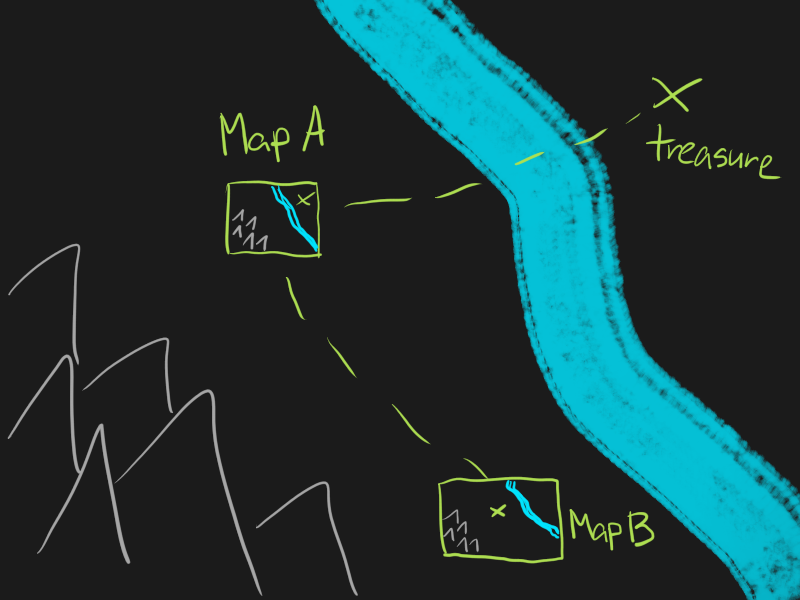Applying the analogy to C - you can create a pointer that contains the address of a pointer to a variable:

``````int treasure = 777;
int* map_A = &treasure;
int** map_B = &map_A;
printf("coordinates in map_B: %p\n", map_B);
// coordinates in map_B: 0x7ffe82475c28
printf("coordinates of map_A: %p\n", &map_A);
// coordinates of map_A: 0x7ffe82475c28
printf("coordinates in map_A: %p\n", *map_B);
// coordinates in map_A: 0x7ffe82475c24
printf("coordinates of treasure: %p\n", &treasure);
// coordinates of treasure: 0x7ffe82475c24
printf("treasure: %d\n", **map_B);
// treasure: 777
``````

This last line, where “**map_B” is written, is the hardest one. Here, we go to the coordinates from the map “B”, we find the map “A”, and already on the map “A”, we get the treasure.

# Different types of pointers

Here already my analogies will start to crack at the seams, because in the C language pointers can be of different types.

Therefore, let me explain here without analogies, but with a visual explanation.

### Variable piece

Let’s say you have an int pointer to an int variable – what if you have a char pointer to an int variable?

``````int treasure = 777;
int* int_treasure_map = &treasure;
// Explicit casting of int* to char* so that the compiler doesn't swear
char* char_treasure_map = (char*)&treasure;
printf("treasure: %d\n", treasure);
// treasure: 777
printf("value by int_treasure_map: %d\n", *int_treasure_map);
// value by int_treasure_map: 777
printf("value by char_treasure_map: %d\n", *char_treasure_map);
// value by char_treasure_map: 9
``````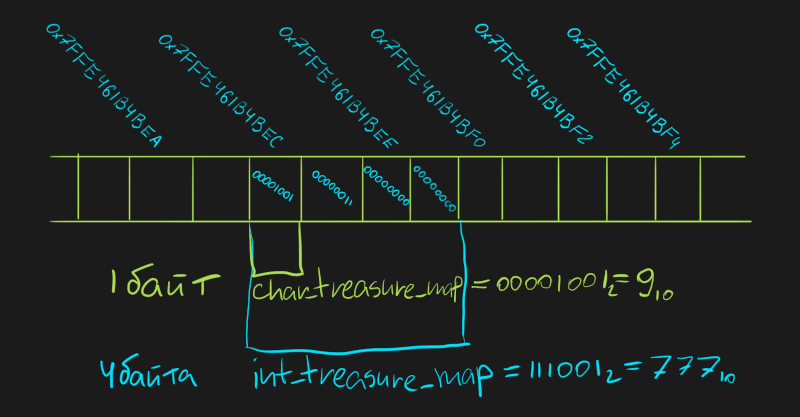Remember how I mentioned byte order before? In this example, we saw live what it is.

It turned out that char_treasure_map returned us the value of the first byte of the int variable treasure. Why only the first byte? Because it’s a pointer to char, and char takes 1 byte.

If I had the byte order not “from low to high”, but “from high to low”, then the value 0 would be returned to me, since the first bytes of the number were high bytes (and there the first two bytes are zeros).

### Wrong variable type

You can also take a float variable, create a pointer to an int, and point it to a float variable:

``````float x = 777;
int* p_x = (int*)&x;
printf("Interpret x as float: %f\nInterpret x as int: %d\n", x, *p_x);
// Interpret x as float: 777.000000
// Interpret x as int: 1145192448

*p_x = 777;
printf("Interpret x as float: %f\nInterpret x as int: %d\n", x, *p_x);
// Interpret x as float: 0.000000
// Interpret x as int: 777
``````

Initially, we assigned the value 777 to the “x” variable as a float variable - it was written into memory in the real representation. Then, we assigned the value 777 to the int pointer “p_x” – to where “p_x” points (to the variable “x”) we wrote it in integer representation.

Here is a visual demonstration of what different representations of the number 777 look like: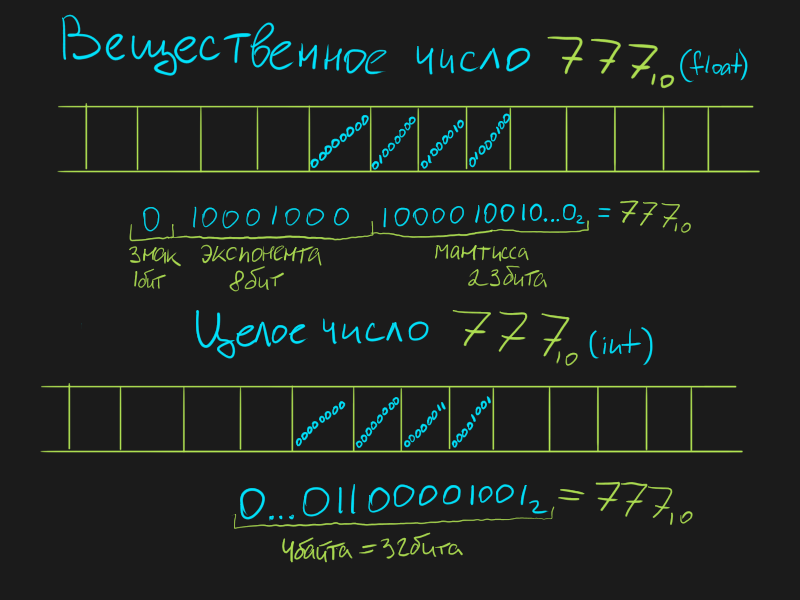If we interpret the real record of a number as an integer, or an integer as a real one, we will not be able to read the value we need normally. It is as if we started reading a book in Czech in Russian, and vice versa - we will understand something, but the meaning of the words will be different.

What I have shown are exotic cases and are not found anywhere in practice. Don’t ever do that!

Always make sure that the types of pointers and variables match, and the correct addresses are written in the pointers.

### Type void

In addition to the types already known to you (char, short int, int, float, double), a pointer can also be a void type. Its features:

• Needed to store the ardes of a variable, regardless of its type
• A void pointer cannot be dereferenced – you can only read the address

Here is an example using void:

``````int x = 777;
void* p = (void*)&x;
printf("address in void pointer: %p\n", p);
// You can't dereference it, but we can first
// cast to the desired type, and then dereference
printf("value by void pointer: %d\n", *((int*)p));
``````

Addresses in pointers can be not only stored, but also somehow changed - for example, to shift the pointer to adjacent cells.

Let me take the previous example and show you what it looks like:

``````int treasure = 777;
int* int_treasure_map = &treasure;
char* char_treasure_map = ((char*)&treasure);
printf("treasure: %d\n", treasure);
// treasure: 777
printf("value by int_treasure_map: %d\n", *int_treasure_map);
// value by int_treasure_map: 777
printf("value by char_treasure_map+0: %d\n", *char_treasure_map);
// value by char_treasure_map+0: 9
printf("value by char_treasure_map+1: %d\n", *(char_treasure_map+1));
// value by char_treasure_map+1: 3
// And I can also shove it into a variable
char* char_treasure_map_2 = ((char*)&treasure) + 2;
printf("value by char_treasure_map+2: %d\n", *char_treasure_map_2);
// value by char_treasure_map+2: 0
// And I can also move forward 1 like this
char_treasure_map_2++;
printf("value by char_treasure_map+3: %d\n", *char_treasure_map_2);
// value by char_treasure_map+3: 0
// And I can move in the opposite direction
char_treasure_map_2 -= 3;
printf("value by char_treasure_map+0: %d\n", *char_treasure_map_2);
// value by char_treasure_map+0: 9
``````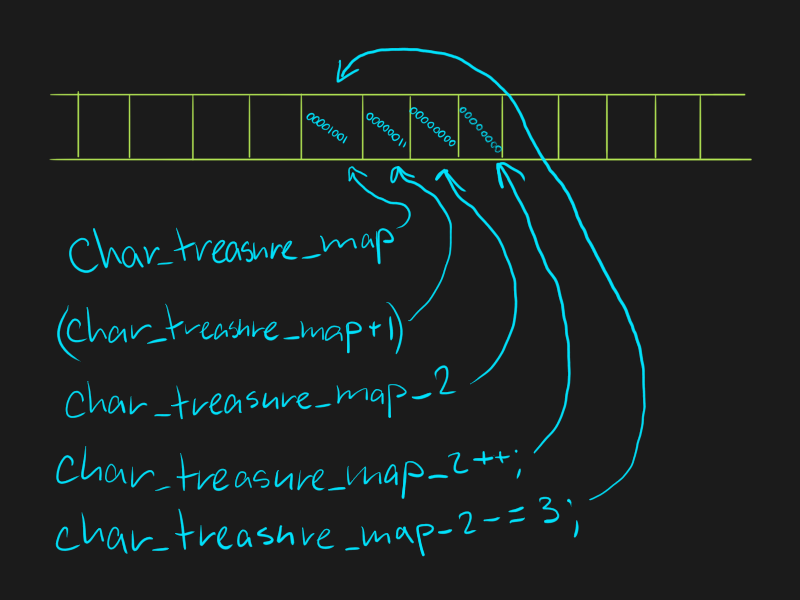If you add/subtract one from the pointer to char, then the address will change to 1, and if you add/subtract one from the pointer to int, then the address will change to 4. Why is this happening?

This is where pointer types come in - depending on the pointer type, the offset occurs by a number of bytes equal to the size of the value of this type. That is, for:

• void and char are shifted by 1 byte
• short int shift by 2 bytes
• int and float are shifted by 4 bytes
• double shifted by 8 bytes

You can also compare them with each other:

``````int a, b;
int *p_a = &a, *p_b = &b;
printf("a: %p\nb: %p\n", p_a, p_b);
if (p_a < p_b)
else
``````

We get this output:

``````a: 0x7ffca02f6860
b: 0x7ffca02f6864
``````

Try to run on your own, most likely you will have the opposite - the address “a” will be greater than the address “b”.

Why? I have a Linux operating system, and on it the addresses, when creating new variables, grow from smaller to larger. That is, first, cells for the variable “a” are allocated in memory, and then for the variable “b”, new cells are allocated to the right of “a”.

You most likely have Windows - in it, when creating variables, addresses grow in the opposite direction, from large to small. That is, first the cells for the variable “a” are allocated in memory, and then for the variable “b”, new cells are allocated to the left of the cell “a”.

# Conclusion

In total, you learned what is:

• Index (map)